FAIL (the browser should render some flash content, not this).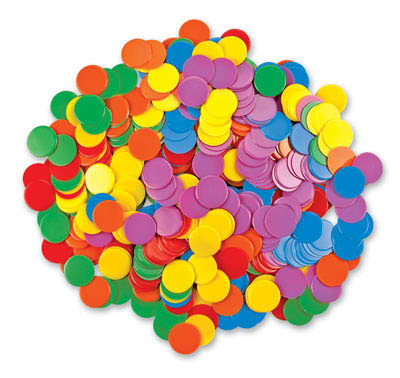Math 7 Honors Ch 1

Sol

1.1
1.2
1.3
1.4
1.5
1.6

Basic Multiplication Skills

• Developing Basic Skills in Multiplying Single Digits, Double Digits
• Apply Skills in Multiplications to Solve Challenging Problems
• Refine Student skills in Multiplication Strategies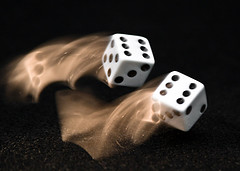Math 7 Honors Ch 2

2.1
2.2
2.3
2.4

Operations with Integers

• These are basic assignments on Adding and Subtracting Fractions
• Although Operations with Fractions are not in the new Gr7 Curriculum, we believe a thorough understanding in fractions would benefit students entering an honours program
• It is recommended that students master the skills required for basic operations of fractions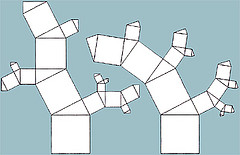Math 7 Honors Ch 3

Q17
Q18

Q19
Q20
Operations with Decimals

In this chapter, students will learn:

• This section deals with rounding numbers to different place values, ie: tens, hundredth, or thousandths place
• Students should be able to add numbers with different place values
• We've also included some challenge problems from Math ChallengersMath 7 Honors Ch 4

Q21
Q22

Q23
Q24

Decimals Fractions and Percentages

In this section, students will learn:

• how to convert numbers from fractions to decimals and vice versa
• find angles involving parallel lines, tangents, and secants
• how to find the percentage of a number
• We've also included some challenge problems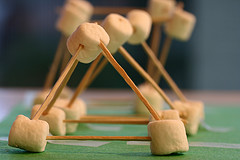Math 7 Honors Ch 5

• Unit 5-1 Fractals
• Problem of the week 25
• Unit 5-2 Fractals
• Problem of the week 26
• Unit 5-3 Fractals
• Problem of the week 27
• Unit 5-4 Fractals
• Problem of the week 28

PDF
PDF
PDF
PDF
Linear Relations Graphs and Tables

In this section, students will learn:

• to make a table of values with an equation
• find a pair of coordinates and plot it on a cartesian plane
• connect the dots of an equation to create a lineMath 7 Honors Ch 6

PDF
PDF
PDF
PDF
PDF

Two Step Equations
Many students entering the honours program have a legitamite level of problem solving skills but lack the basic knowledge of algebra. Algebra provides an efficient way to solve problems and can be a great tool.
In this section, students will learn:

• to solve an equation that requires one or two steps
• to solve equations that involves fractions and brackets
• to solve word problems using equationsMath 7 Honors Ch 7

PDF

PDF

Areas and Circumferences of Circles

In this section, students will learn:

• to calculate the circumference and areas of circles and shapes that involve part of a circle
• to calculate the radius when given the area or circumference
We've also included some challenge problems from Math Challengers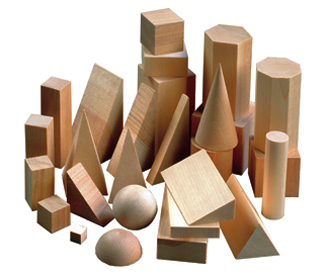Math 7 Honors Ch 8

PDF
PDF
PDF
PDF
Volumes of Cylinders and Rectangular Prisms

In this section, students will learn:

• to find the volume and surface areas of Cylinders, Cones, and various 3D Shapes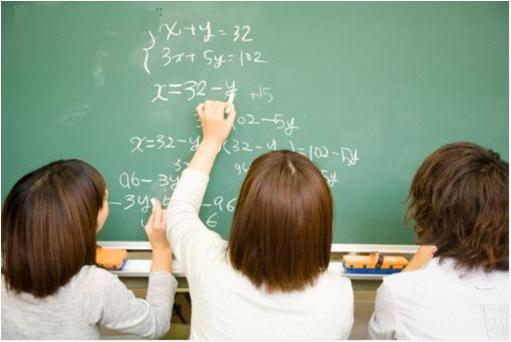Math 7 Honours Ch9
•  2008 Intramurals Sprint
•  2008 Intramurals Target and Team
•  Challenge Problems with Solids

PDF
PDF
PDF
PDF
Grade 7 Honours Past Entrance Exams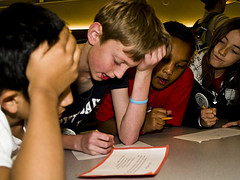Math 7 Honours Ch 10

•  Gr 7 Honours Entrance Exam
•  2011 Regionals Competition
•  2011 Regionals Competition
•  2011 Regionals Competition

SLA
SLB
SLC
SLD
Probability with Independent Events

•  2012 Regionals Competition
•  2012 Provincial Competiton
•  2013 Regionals Competition
•  2013 Provincials Competition